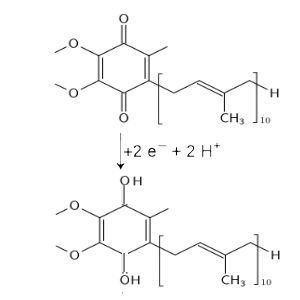﻿Search:

# The electron transport chain (respiratory chain)

The citric acid cycle (Krebs cycle) produces $NADH$ nicotinaminedinucleotide and $FADH_2$ flavineadeninedinucleotide. The respiratory chain (electron transport chain) is the "chain" of the complex reactions of these two species with inhaled $O_2$ oxygen leading to the transformation of the $ADP$ into $ATP$ (thus to the "energy recharge of the body" )

## Summary (simplified)

$\frac{1}{2}O_2+2H^++2e^-$ → $H_2O$ $NADH-2e^-$ → $NAD^+ + H^+$ ___________________________________________ $\frac{1}{2}O_2+NADH + H^+$ → $NAD^+ + H_2O$ - 3 ATP produced -

$\frac{1}{2}O_2+2H^++2e^-$ → $H_2O$ $FADH_2-2e^-$ → $FAD + 2H^+$ ___________________________________________ $\frac{1}{2}O_2+FADH_2$ → $FAD + H_2O$ - 2 ATP produced -

## Details (still simplified)

### $NADH$

The transformations take place in the inner membrane of the mitochondria.I (Oxidation): $NADH-2e^-$ → $NAD^+ + H^+ + 2e^-$ II: (Reduction):Ubiquinone (a diketone) is reduced to ubiquinol (a paradiphenol):III et IV: (Oxidation-reduction) Two complex enzymes (an oxidase and a reductase) associated with cytochrome c reoxidize ubiquinol to ubiquinone while transferring electrons to $\frac{1}{2}O_2$ which is reduced to $H_2O$ During these complex transformations, three additional $H^+$ ions are released in the intermembrane space.

### $FADH_2$

The oxidation also takes place in the inner wall of the mitochondria: $FADH_2-2e^-$ → $FAD + 2H^+$ Subsequent transformations are similar to those for $NADH$ except that only two additional $H^+$ ions are released in the intermembrane space.

### $ATP$

Previous transformations create a $H^+$ ion gradient between the intermembrane space and the internal mitochondrial matrix. The ATPsynthetase enzymes located in the inner membrane allow the passage of these ions to the matrix. The energy released by the reduction of this $H^+$ ion gradient serves to couple the phosphate ions (for each $H^+$ ion one phosphate ion abbreviated by $P_i$) to the $ADP$ in order to synthesize $ATP$: $ADP+P_i$ → $ATP$

## Reférences

For details on the role of the NAD+ see →   this For details on the role of the FAD see →   this For the Krebs cycle look at →   this